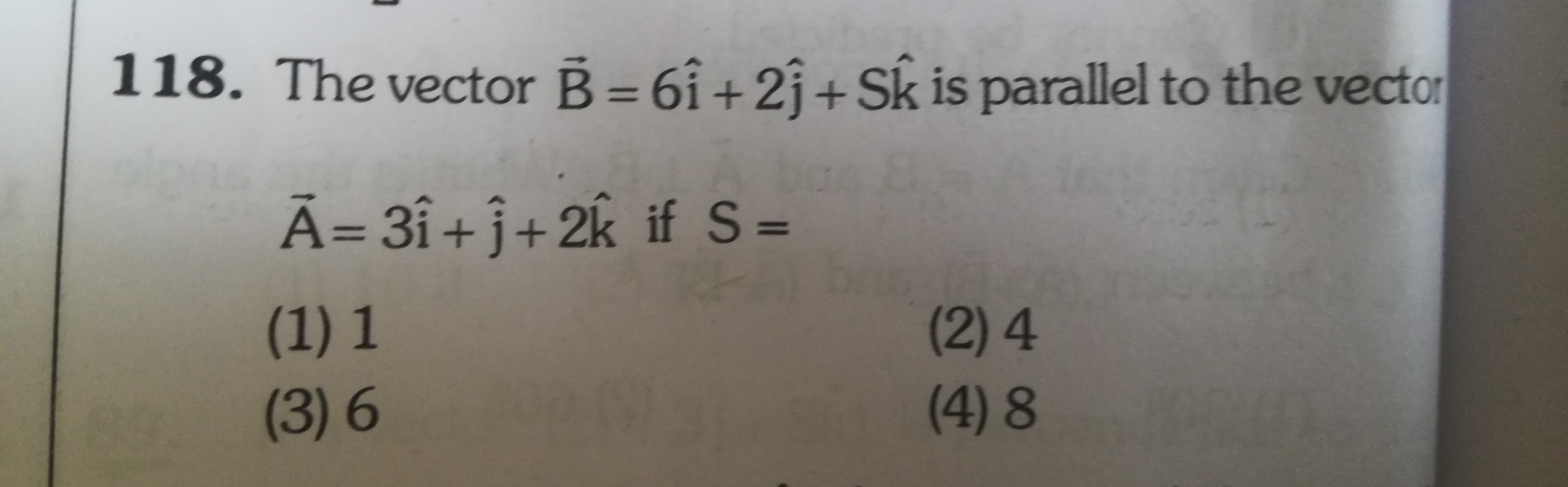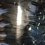# vector questionHi, can anyone explain the answer? thanksNote by Shruthi Srinivasan
1 year ago

This discussion board is a place to discuss our Daily Challenges and the math and science related to those challenges. Explanations are more than just a solution — they should explain the steps and thinking strategies that you used to obtain the solution. Comments should further the discussion of math and science.

When posting on Brilliant:

• Use the emojis to react to an explanation, whether you're congratulating a job well done , or just really confused .
• Ask specific questions about the challenge or the steps in somebody's explanation. Well-posed questions can add a lot to the discussion, but posting "I don't understand!" doesn't help anyone.
• Try to contribute something new to the discussion, whether it is an extension, generalization or other idea related to the challenge.

MarkdownAppears as
*italics* or _italics_ italics
**bold** or __bold__ bold
- bulleted- list
• bulleted
• list
1. numbered2. list
1. numbered
2. list
Note: you must add a full line of space before and after lists for them to show up correctly
paragraph 1paragraph 2

paragraph 1

paragraph 2

[example link](https://brilliant.org)example link
> This is a quote
This is a quote
    # I indented these lines
# 4 spaces, and now they show
# up as a code block.

print "hello world"
# I indented these lines
# 4 spaces, and now they show
# up as a code block.

print "hello world"
MathAppears as
Remember to wrap math in $$ ... $$ or $ ... $ to ensure proper formatting.
2 \times 3 $2 \times 3$
2^{34} $2^{34}$
a_{i-1} $a_{i-1}$
\frac{2}{3} $\frac{2}{3}$
\sqrt{2} $\sqrt{2}$
\sum_{i=1}^3 $\sum_{i=1}^3$
\sin \theta $\sin \theta$
\boxed{123} $\boxed{123}$

Sort by:

Ok, I'm going to give a more intuitive answer:

The vector $\vec{A}$ is $3 \hat{i} + \hat{j} + 2 \hat{k}$.

Any vector that is parallel to $\vec{A}$ has to point in the same direction.

Now, here's the trick; for the vector $\vec{B}$ to point in the same direction, its components have to be the same.

However, they don't have to be the same vector; $\vec{B}$ can be any scaled version of $\vec{A}$ and still point in the same direction.

So, knowing this, we see that $\vec{B}$ has first two components twice as much as $\vec{A}$. For it to maintain the property of a scaled version of $\vec{A}$, it must have the 3rd component to be twice as much as the 3rd component of $\vec{A}$.

Therefore $\boxed{S = 4}$

The main point this intuitive solution makes is that for a vector to be parallel to another vector, it must point in the same direction as the second vector, and for that to happen numerically, it has to have any scaled version of vector 2's components.

- 11 months, 3 weeks ago

($2$) - $4$ because:

$2A = B$

So:

$2(3i + j + 2k) = B$

$6i + 2j + 4k = B$

$S = \fbox 4$

or:

$A =$$\frac{1}{2}$$B$

So:

$\frac{S}{2}$$= 2$

$S = \fbox 4$

- 1 year ago

thank you.

- 1 year ago

You're welcome!

- 1 year ago

That's not technically right. Just because they are parallel, that doesn't mean that $2A = B$. Why not $A = \tfrac12 B$?

- 1 year ago

That's also true.

- 1 year ago

You're missing the point. Why must one of the vector be double of the other vector?

- 1 year ago